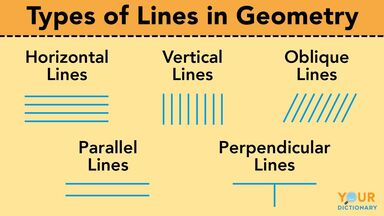# Types of Lines in Geometry: Examples Anyone Can Understand

A line is defined as a one-dimensional geometric figure with length but no width. It extends infinitely in either direction with no ends, and the equation of a straight line is ax + b = 0. Keep reading to learn about the five main types of lines in geometry with line examples in your everyday life.types of lines in geometry

## Horizontal Lines

A horizontal line goes from left to right. It runs along the x-axis without touching any point. Examples of horizontal lines include the line between the ocean and the sky (the horizon), lines on a sheet of notebook paper and the edge of a table.

## Vertical Lines

Vertical lines are straight lines that go up and down. They run along the y-axis, and like horizontal lines, they never touch a point on the axis. You'll see vertical lines in architectural pillars, the trunks of trees and the lines of a skyscraper.

## Oblique Lines

Straight lines that are not horizontal or vertical are called oblique lines. These lines do cross the x and y-axis. When an oblique line joins two vertices of a polygon, it's called a diagonal line.

## Parallel Lines

When horizontal, vertical or oblique lines run next to each other without ever meeting or intersecting, they are parallel lines. These lines are always the same distance apart (also known as equidistant) and stretch into infinity. The symbol for parallel lines is ||, and you can find them in striped clothing, fence posts or any other series of lines.

## Perpendicular Lines

Perpendicular lines occur when a vertical line and a horizontal line cross each other and create a 90° angle (also known as a right angle). The symbol for perpendicular lines is ⊥, and you're most likely to see them on a grid or where lines come together to make a T shape.

## Other Geometric Lines

So far, you've learned about five different types of straight lines. However, there are more types of lines that you'll find in geometry. It's important to know what type of line you're looking at so you can measure and calculate it properly.

• intersecting lines - lines that meet at a point at any angle (unlike perpendicular lines, which only meet at 90°)
• secant lines - lines that intersect a curve at two or more points
• skew lines - lines that have no intersections with each other but are not equidistant (unlike parallel lines)
• tangent lines - lines that touch a curve at one point

Older math curriculum names a curved line as a line. However, because graphing a linear function results in a straight line, many mathematicians consider a curve not to be a line, as a curve on a graph is derived from a non-linear function.

### Line Segments and Rays

Some people get confused about the differences between lines, line segments and rays. Basically, the differences in their meanings are:

• line - continues for infinity
• line segment - has two endpoints
• ray - has one endpoint; the other side continues for infinity

You can measure the length of a line segment, which is a part of a line, and all the points inside. You can't measure a line or a ray, as they each have at least one side that continues without an endpoint.

## Lining It Up in Mathematics

Learning what different types of lines exist in geometry can help you understand more about your world. Once you know how these lines work, you expand your geometric knowledge. Take a look at the different types of angles to learn what happens when lines or rays intersect at certain points. Or, check out the ten major types of graphs and how they factor into your math curriculum.